# Online Civil Engineering Test - Engineering Economy TestLoading Test...

Instruction:

• This is a FREE online test. DO NOT pay money to anyone to attend this test.
• Total number of questions : 20.
• Time alloted : 30 minutes.
• Each question carry 1 mark, no negative marks.
• DO NOT refresh the page.
• All the best :-).

1.

The ratio obtained by dividing 'quick assests' by current liabilities is called

A.
 Turnover ratioB.
 Acid test ratioC.
 Solvency ratioD.
 None of these.2.

Which one of the following definitions, is correct ?

A.
 The ratio of total debt to share holder's equity is called 'debt ratio'.B.
 The ratio debt-to-total assests is called Debt-to-total assest ratio.C.
 The ratio of earnings before interest and taxes for a particular reporting period to the amount of interest charges for the period, is called interest coverage ratio.D.
 All of these3.

Pick up the element of the cost from the following:

A.
 direct materialB.
 direct labourC.
 Over headD.
 All of these4.

Pick up the correct statement from the following:

A.
 The change in the amount of money over a given time period is called 'time value' of money, a most important concept in engineering economy.B.
 The manifestation of the time value of money is termed as interest.C.
 Interest on borrowing = present amount owed - original loanD.
 The original investment (or loan) is referred to as principal.E.
 All of these.5.

In the cash-flow diagram shown in the given figure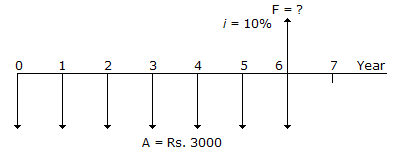A.
 Equal deposits of Rs 3000 per year (A) are made, starting now.B.
 The rate of interest is 10% per yearaccountC.
 The amount accumulated after the seventh deposit is to be computedD.
 All of these6.

The estimate based on a detailed quantity survey and furnishes the most accurate and reliable estimate possible is known as

A.
 Conceptual estimateB.
 Definitive estimateC.
 Probabilistic estimateD.
 None of these7.

Current assests less inventories divided by current liabilities is known as

A.
 Liquidity ratioB.
 Current ratioC.
 Acid-Test (or Quick) ratioD.
 Debts ratio8.

If a is the base amount expenditure, b is the increase in the operation cost each year over a period of n years, the total cost of maintenance is :

A.
 a + (n + 1) bB.
 a + (n - 1) bC.
 a x (n - 1) bD.
 a - (n - 1) b9.

In a cash flow series :

A.
 uniform gradient signifies that an income or disbursement changes by the same amount in each interest period.B.
 Either an increase or a decrease in the amount of a cash flow is called the gradient.C.
 The gradient in the cash flow may be positive or negative.D.
 All of these10.

The capital Recovery Factor (equal payments) of Capital Recovery Annuity is :

A.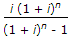B.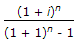C.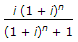D.
 None of these.11.

The interest calculated on the basis of 365 days a year, is known as :

A.
 interestB.
 ordinary simple interestC.
 exact simple interestD.
 None of these12.

Pick up the correct reason for making conceptual (or preliminary) estimate from the following:

A.
 To have a check on a definitive cost estimate.B.
 To check qoutations from contractors and/or sub contractors.C.
 To compute target estimate for the owner while drawings and specifications are in initial stage.D.
 All of these.13.

Pick up the correct statement from the following:

A.
 A NPV profile graph shows the curvilinear relationship between the net present value of the project and discount rate employed.B.
 In a NPV profile, if discount rate is zero, then net present value is simply total cash inflows less the total cash outflows of the project.C.
 As the discount rate increases, the net present value profile slopes downward to the right.D.
 All of these14.

If a seller recovers his capital along with accumulated compensating interest not in one single lumpsum payment but in periodical equal payments, over time :

A.
 Capital Recovery Annuity fs availedB.
 Present work Annuity is availedC.
 Sinking Fund Annuity is availedD.
 Sinking Fund Annuity is availed15.

The financial analysis :

A.
 helps a share holder to compare the expected return on his investment in the firm against the expected return from other alternative investment.B.
 helps a bank to know the financial position of the firm for granting a loan to the firm.C.
 helps to judge the success of the firm's financial plans.D.
 All of these.16.

Pick up the correct statement regarding financial statement analysis from the following.

A.
 Final analysis always involves the use of various financial statements i.e., balance sheet and income statement.B.
 The balance sheet is the summary of assests, liabilities and owner's equity of business at a point in time.C.
 The income statement is the summary of revenues and expenses of a firm over a particular period of time.D.
 All the above17.

In the cash flow diagram shown in the given figure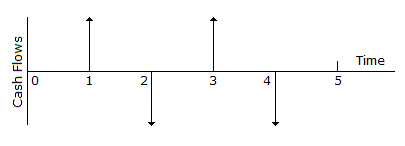A.
 The first disbursement occurs at the end of year 2B.
 The second disbursement occurs at the end of year 4C.
 The first receipt occurs at the end of year 1D.
 The second receipt occurs at the end of year 3E.
 All of these18.

The construction manager uses the estimate of the project

A.
 to tell the owner of the project to take his/her financial decision.B.
 to advise the architect/engineer regarding design cost parameter especially in value engineering analysis.C.
 to develop bids on the project.D.
 to control the project during its construction.E.
 All of these19.

Liquidity ratios are used :

A.
 to measure a firms ability to meet short-cut obligations.B.
 to compare short term obligations to short-term resources available to meet these obligations.C.
 to obtain much insight into the present cash solvency of the firm and the firm's ability to remain solvent in the event of adversity.D.
 All of these20.

Pick up the correct statement from the following:

A.
 The difference between sales revenue and cost of goods sold, is known as 'Gross Profit.'B.
 The gross profit percentage is the average profit margin obtained on goods sold.C.
 The relationship of contribution to sales is known as contribution ratioD.
 The difference between sales and variable cost of sales, is called contribution.E.
 All of these